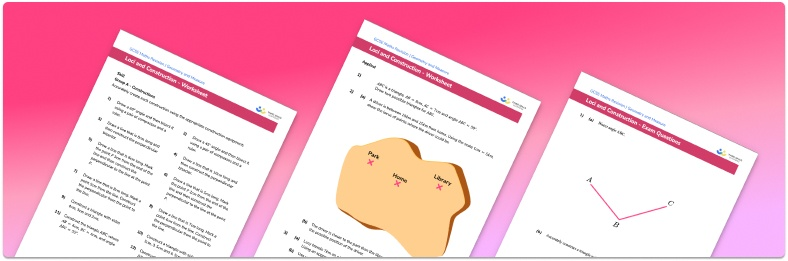# Loci and construction Worksheet• Section 1 of the loci worksheet contains 20+ skills-based loci and construction questions, in 3 groups to support differentiation
• Section 2 contains 4 applied loci and construction questions with a mix of worded problems and deeper problem solving questions
• Section 3 contains 4 foundation and higher level GCSE exam style loci and construction questions
• Answers and a mark scheme for all loci and construction questions are provided
• Questions follow variation theory with plenty of opportunities for students to work independently at their own level
• All questions created by fully qualified expert secondary maths teachers
• Suitable for GCSE maths revision for AQA, OCR and Edexcel exam boards

• This field is for validation purposes and should be left unchanged.

You can unsubscribe at any time (each email we send will contain an easy way to unsubscribe). To find out more about how we use your data, see our privacy policy.

### Loci and construction at a glance

There are a number of useful constructions which can be drawn using a ruler, a protractor and a pair of compasses. These include constructing triangles, constructing an angle bisector, constructing the perpendicular bisector of a line segment and constructing the perpendicular line at a given point p. We need to use the most efficient method to draw each construction, and we must leave construction lines on the diagram.

Constructions are useful when drawing loci. A locus of points is a set of points that follow a certain rule. For example, the set of points that are exactly 2m from an object A would be any point that lies on the circle with a radius of 2m, centred at A. Another example is the set of points that are equidistant (the same distance) from two lines which meet at a point (an angle). When we bisect an angle we cut it exactly in half, and so the straight line that bisects the angle would indicate all of the points that are equidistant from the two lines.

When constructing triangles, we need to know at least 3 pieces of information about the triangle’s angles and side lengths. If we use S to represent a side length, and A to represent an angle, we can construct triangles of the form ASA (two angles and a side length known), SSS (three sides known) or SAS (two sides and one angle known). We cannot construct a triangle when we only know three angles; we must know at least one side length. You should be familiar with the four main types of triangle: equilateral triangles, isosceles triangles, scalene triangles, and right angle triangles.

Looking forward, students can then progress to additional geometry worksheets, for example a 3D shapes worksheet or area of a triangle worksheet.For more teaching and learning support on Geometry our GCSE maths lessons provide step by step support for all GCSE maths concepts.

## Do you have KS4 students who need more focused attention to succeed at GCSE?There will be students in your class who require individual attention to help them succeed in their maths GCSEs. In a class of 30, it’s not always easy to provide.

Help your students feel confident with exam-style questions and the strategies they’ll need to answer them correctly with our dedicated GCSE maths revision programme.

Lessons are selected to provide support where each student needs it most, and specially-trained GCSE maths tutors adapt the pitch and pace of each lesson. This ensures a personalised revision programme that raises grades and boosts confidence.

Find out more